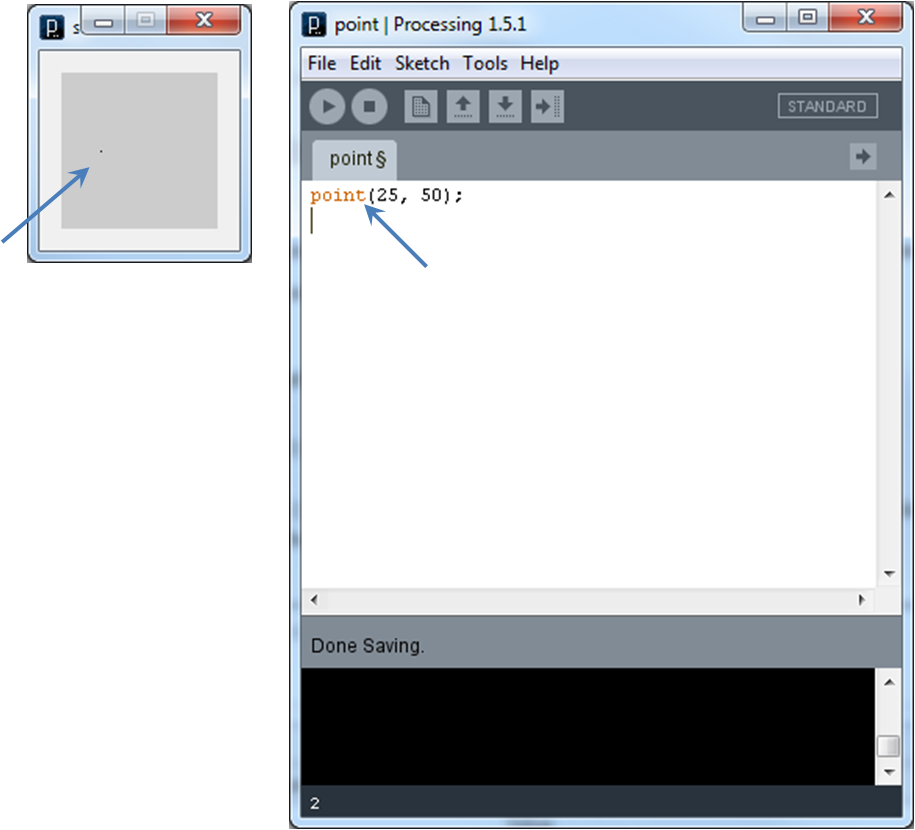Processing provides some predefined methods, which are predefined blocks of code that can be called by naming the method and passing an appropriate list of arguments.

• Pattern:

``` methodName(argumentList); ```
where:
• methodName is the predefined name (also called an identifier) of a particular method.
• argumentList is a list (possible empty) of items called arguments expected by the method that are in a specified order, enclosed in parentheses, and separated by commas.
• As an example, consider the `point()` method, which is predefined by Processing:

• Pattern for the `point()` method:

``` point(xPosition, yPosition); ```
where:
• xPosition is the x coordinate of the screen pixel where the point should be centered, and
• yPosition is the y coordinate of this same screen pixel.
• Calling the `point()` method:

`point(25, 50);`
Here, the method `point()` is called, passing two literal arguments, `25` and `50`, which specify the x and y coordinates of the point being drawn respectively.